Ifor, where f and g are differentiable andexists, this limit coincides with.To apply the L'Hôpital's rule, there must be a limit in the form, where a can be a number or infinite, and have the indeterminate forms: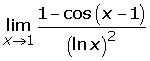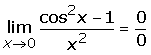Infinity Minus Infinity

For the indeterminate form of infinity minus infinity, the fractions put themselves into a common denominator.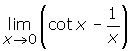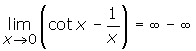Zero Times Infinity

The indeterminate form of zero times infinity is transformed as follows:Indeterminate FormsIn the indeterminate forms zero to the power of zero, infinity to the power of zero and one to the power of infinity; make the following transformations: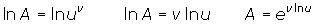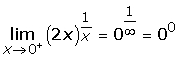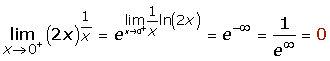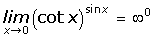Did you like the article?(No Ratings Yet)Loading...

Emma

Did you like
this resource?

Bravo!